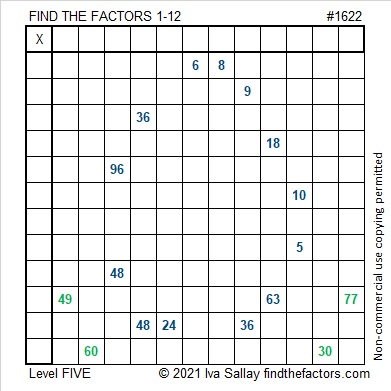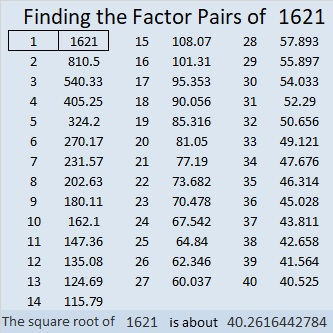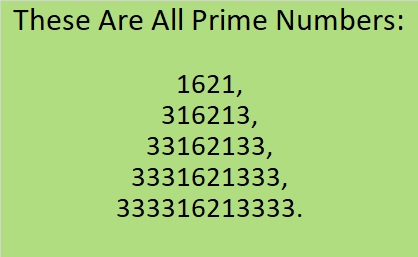### Today’s Puzzle:

These somewhat tricky level-5 puzzles are probably better suited for middle school and up than younger kids. Use logic on every step and you should be able to find its unique solution.Here are some Easter egg puzzles I saw on Twitter. Some are perfect for the littles and others are for older kids. Easter egg hunts can be fun for anyone of any age.

### Factors of 1622:

• 1622 is a composite number.
• Prime factorization: 1622 = 2 × 811.
• 1622 has no exponents greater than 1 in its prime factorization, so √1622 cannot be simplified.
• The exponents in the prime factorization are 1 and 1. Adding one to each exponent and multiplying we get (1 + 1)(1 + 1) = 2 × 2 = 4. Therefore 1622 has exactly 4 factors.
• The factors of 1622 are outlined with their factor pair partners in the graphic below.### More about the Number 1622:

1622 is the sum of four consecutive numbers:
409 + 410 + 411 + 412 = 1622.

# 1621 Give This Purple Egg a Crack!

### Today’s Puzzle:

Here’s a level-5 purple Easter egg for you to try. All you need to do is write the numbers from 1 to 12 in both the first column and in the top row so that those numbers and the given clues function like a multiplication table. Go ahead. Give it a crack!### Factors of 1621:

• 1621 is a prime number.
• Prime factorization: 1621 is prime.
• 1621 has no exponents greater than 1 in its prime factorization, so √1621 cannot be simplified.
• The exponent in the prime factorization is 1. Adding one to that exponent we get (1 + 1) = 2. Therefore 1621 has exactly 2 factors.
• The factors of 1621 are outlined with their factor pair partners in the graphic below.How do we know that 1621 is a prime number? If 1621 were not a prime number, then it would be divisible by at least one prime number less than or equal to √1621. Since 1621 cannot be divided evenly by 2, 3, 5, 7, 11, 13, 17, 19, 23, 29, 31, or 37, we know that 1621 is a prime number.

### More about the Number 1621:

OEIS.org informs us that 1621 is in an interesting group of prime numbers.I have verified it. They really are all prime!

1621 is the sum of two squares:
39² + 10² = 1621.

1621 is the hypotenuse of a Pythagorean triple:
780-1421-1621, calculated from 2(39)(10), 39² – 10², 39² + 10².

Here’s another way we know that 1621 is a prime number: Since its last two digits divided by 4 leave a remainder of 1, and 39² + 10² = 1621 with 39 and 10 having no common prime factors, 1621 will be prime unless it is divisible by a prime number Pythagorean triple hypotenuse less than or equal to √1621. Since 1621 is not divisible by 5, 13, 17, 29, or 37, we know that 1621 is a prime number.